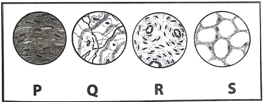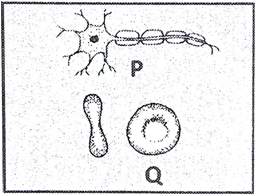# SOF - NSO Level 2 Sample Papers for Class 9

Resources:

Class 9 sample paper & practice questions for the level 2 of National Science Olympiad (NSO) are given below. Syllabus for level 2 is also mentioned for these exams. You can refer these sample paper & quiz for preparing for the exam.Q.1 Q.2 Q.3 Q.4 Q.5 Q.6 Q.7 Q.8 Q.9 Q.10
 Q.1 Read the given statements and mark the correct option. Statement 1: Any two bodies in the universe attract each other. Statement 2: Mass of a body keeps changing with the place, in the universe. a) Both statements 1 and 2 are true and statement 2 is the correct explanation of statement 1. b) Both statements 1 and 2 are true but statement 2 is not the correct explanation of statement 1. c) Statement 1 is true but Statement 2 is false. d) Statement 1 is false but statement 2 is true.
 Q.2 Which of the following structures helps you to determine that it is not an animal cell? Cell wall and vacuole Mitochondria and ribosome Ribosomes and lysosomes Plasma membrane a) Only 1 b) Only 2 c) Both 2 and 4 d) Both 1 and 3
 Q.3 Two flasks I and II contain oxygen (O2)and ozone (O3) respectively. Flask I contains 0.5 moles of oxygen while flask II contains 0.4 moles of ozone. Which of the two flasks have a greater number of oxygen atoms? a) Flask II containing ozone has a greater number of oxygen atoms as compared to flask I. b) Flask I containing oxygen has a greater number of oxygen atoms as compared to flask II. c) Both flasks I and II have the same number of oxygen atoms. d) The number of atoms of oxygen cannot be calculated from the available data.
 Q.4 If the density of air at a point through which a sound wave is passing is maximum at an instant, what will be the pressure at that point? a) Minimum b) Equal to the density of air c) Equal to the atmospheric pressure d) Maximum
 Q.5 At what temperature should all the gases occupy zero volume? a) 0oC b) -273oC c) 273oC d) 100oC
 Q.6 Which of the following tissues supports internal organs and helps in the repairing of tissues?a) P b) Q c) R d) S
 Q.7 Which of the following statements is not true about the given figure?a) P and Q have a nucleus b) P and Q carry out life processes c) P and Q are animal cells d) P is a nerve cell
 Q.8 A body weighs 12 N on the surface of the moon. What is its weight on the surface of the earth? a) 72 N b) 2 N c) 24 N d) Zero
 Q.9 How many gram-atoms and atoms are there in 10 grams of calcium? a) 0.25 gram atoms, 6.023 X 10 23 atoms b) 0.25 gram atoms, 1.50 x 10 23 atoms c) 0.1 gram atoms, 6.023 x 10 23 atoms d) 0.1 gram atoms,1.50 x 10 23 atoms
 Q.10 Identify the correct increasing order of molecular weights of the given compounds. a) H2O>CO2>SO2>H2S b) H2O>H2S>CO2>SO2 c) H2O>SO2>H2S>CO2 d) H2S>CO2>SO2>H2O